# 1715 A Lot More of a Subtraction Distraction

### Today’s Puzzle:

Last time I published a puzzle with the last clue missing. Leaving out the first or the last clue only makes the puzzle slightly more difficult. What if I left out a clue more in the middle of the puzzle. I gave that some thought and designed today’s puzzle. I soon realized that I had to let you know that the 12 is one of the last eight boxes. There is only one solution. Can you find it?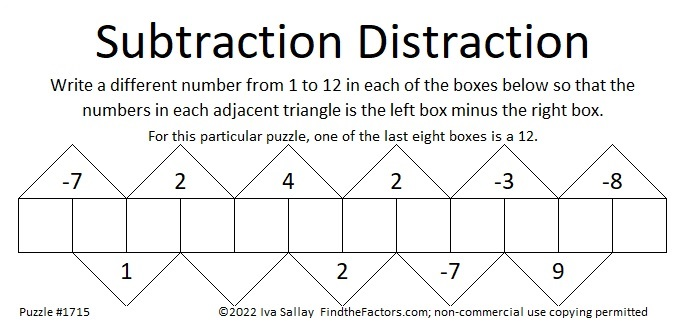I posted a solution video for it on Twitter:

### Factors of 1715:

• 1715 is a composite number.
• Prime factorization: 1715 = 5 × 7 × 7 × 7, which can be written 1715 = 5 × 7³.
• 1715 has at least one exponent greater than 1 in its prime factorization so √1715 can be simplified. Taking the factor pair from the factor pair table below with the largest square number factor, we get √1715 = (√49)(√35) = 7√35.
• The exponents in the prime factorization are 1 and 3. Adding one to each exponent and multiplying we get (1 + 1)(3 + 1) = 2 × 4 = 8. Therefore 1715 has exactly 8 factors.
• The factors of 1715 are outlined with their factor pairs in the graphic below.### More About the number 1715:

1715 = 1·7³·1·5. Thank you, OEIS.org for that fun fact!

1715 is the hypotenuse of a Pythagorean triple:
1029-1372-1715, which is (3-4-5) times 343.

1715 = 5·7³.
5·7º = 2² + 1².
5·7¹ cannot be written as the sum of two squares.
5·7² = 14² + 7².
5·7³ cannot be written as the sum of two squares.
5·7⁴ = 98² + 49².

What do you notice? What do you wonder?

# 1713 A Little More of a Subtraction Distraction

### Today’s Puzzle:

It occurred to me that as long as the last box is neither 1 nor 12 that I could leave the clue above it blank. Can you still solve the puzzle?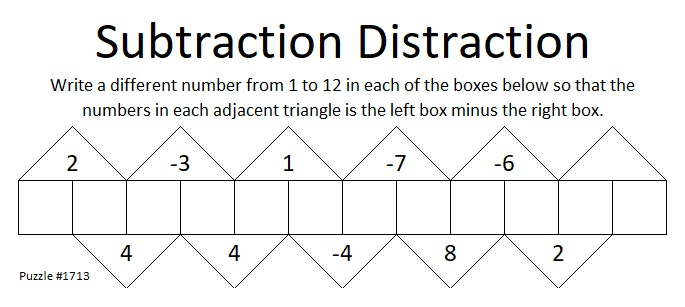### Factors of 1713:

• 1713 is a composite number.
• Prime factorization: 1713 = 3 × 571.
• 1713 has no exponents greater than 1 in its prime factorization, so √1713 cannot be simplified.
• The exponents in the prime factorization are 1 and 1. Adding one to each exponent and multiplying we get (1 + 1)(1 + 1) = 2 × 2 = 4. Therefore 1713 has exactly 4 factors.
• The factors of 1713 are outlined with their factor pair partners in the graphic below.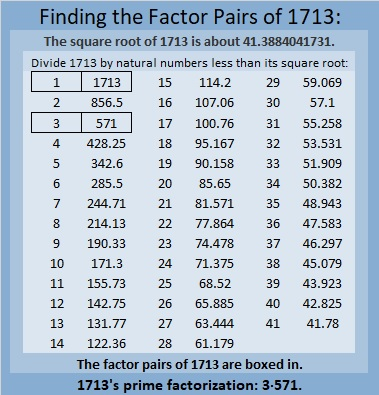### More About the Number 1713:

1713 is the difference of two squares in two different ways:
857² – 856² = 1713, and
287² – 284² = 1713.

1713 is the sum of two, three, and six consecutive numbers:
856 + 857 = 1713,
570 + 571 + 572 = 1713, and
283 + 284 + 285 + 286 + 287 + 288 = 1713.

Do you see any relationship between those two facts?

# 913 Haunted Ten Frame House for Ten Timid Ghosts

Last year right before Halloween, I read a wonderful book to a class of kindergartners. The book was Ten Timid Ghosts by Jennifer O’Connell. The students drew haunted houses and a few trees. As I read the story, they used ten bean counters to show where each ghost was during the story. At any given time each ghost was either in the house or in the woods. They could see lots of number sentences as the story progressed.

This year I liked the idea of making a haunted house with ten window frames and ten sections for the trees in the woods. That way each of the ten ghosts could have a specific place to be throughout the story. I made the ten frames vertical to make odd and even amounts easier to see. There seems to be some ghostly figures flying around the tree branches, too.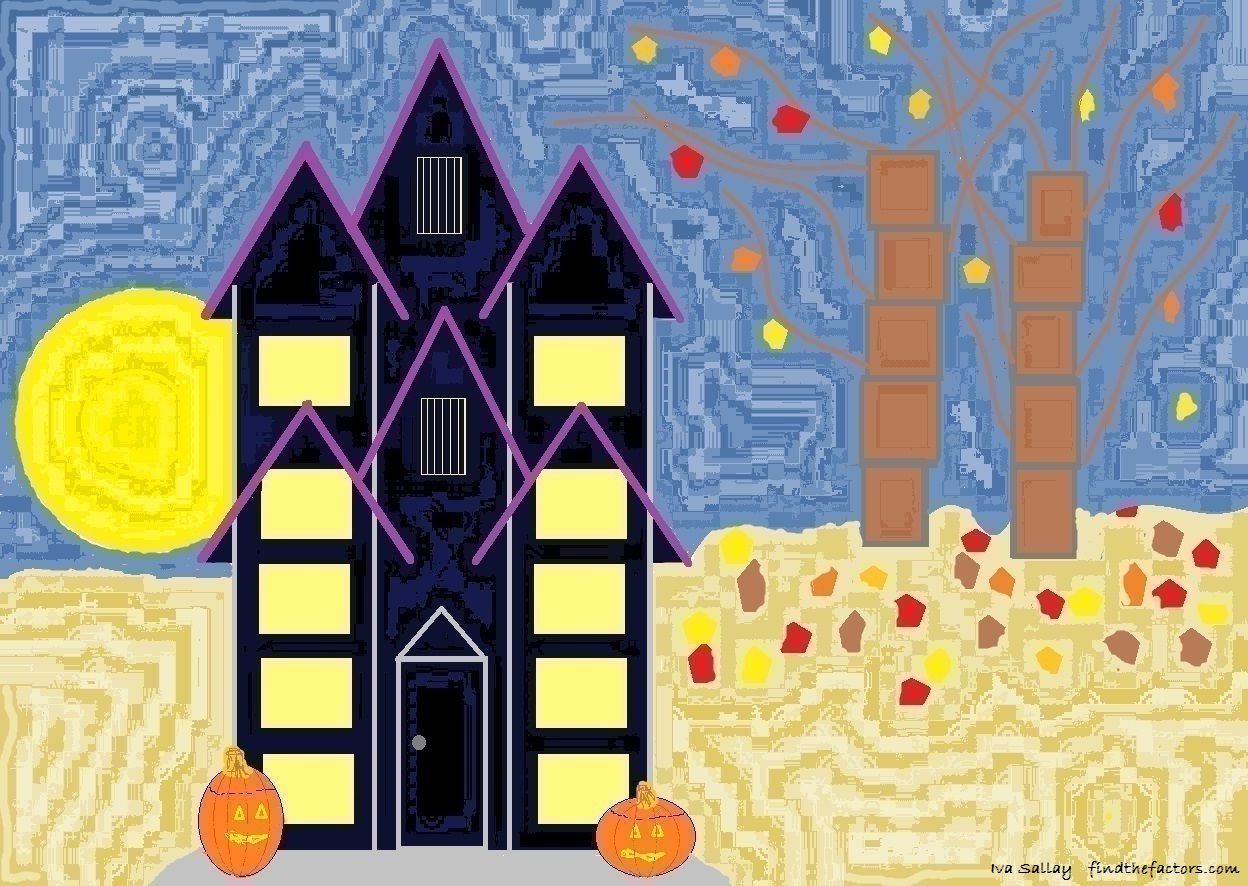You are welcome to use this haunted house as you add and subtract ghosts from the house and the woods. You’ll need some ghosts, too. Dry great northern beans are white and make great ghosts. If you like, you can add eyes and a mouth to the ghostly beans with a marker.

For those who would rather use less printer ink, I’ve made a similar picture that can be colored after it is printed:You may also want to look at another activity that uses this same book. Sara Gast’s power point was especially made for kids with autism, but other children would enjoy it as well. You can use her activity to reinforce the concept of ordinal numbers, too.

Young children everywhere are sure to enjoy this fun book as they learn to count and to add and subtract.

Now I’ll write a little bit about the number 913.

If you’ve read my last few posts, you may have noticed that 910, 911, and 912 can each be represented in BASE 26 using their base ten digits in a different order. That pattern continues for 913. In fact, it is true for 914 – 919, as well. However, 913 is even more special because not only is it 193 in BASE 26, it is also 391 in BASE 16. Thank you, OEIS.org for that fun fact.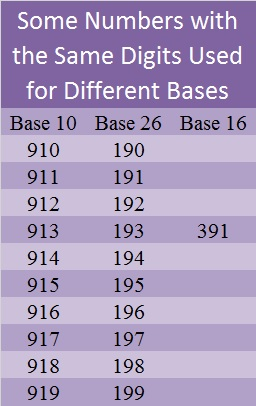9 – 1 + 3 = 11, so 913 is divisible by eleven.

• 913 is a composite number.
• Prime factorization: 913 = 11 × 83
• The exponents in the prime factorization are 1 and 1. Adding one to each and multiplying we get (1 + 1)(1 + 1) = 2 × 2 = 4. Therefore 913 has exactly 4 factors.
• Factors of 913: 1, 11, 83, 913
• Factor pairs: 913 = 1 × 913 or 11 × 83
• 913 has no square factors that allow its square root to be simplified. √913 ≈ 30.2158899# 279 How to Add and Subtract 5 with Secret Identities

• 279 is a composite number.
• Prime factorization: 279 = 3 x 3 x 31, which can be written (3^2) x 31
• The exponents in the prime factorization are 2 and 1. Adding one to each and multiplying we get (2 + 1)(1 + 1) = 3 x 2  = 6. Therefore 279 has 6 factors.
• Factors of 279: 1, 3, 9, 31, 93, 279
• Factor pairs: 279 = 1 x 279, 3 x 93, or 9 x 31
• Taking the factor pair with the largest square number factor, we get √279 = (√9)(√31) = 3√31 ≈ 16.703Do you know who Superman is? I’ve asked many children in their first year of school that question. They know who he is, and they can tell me lots of things about him.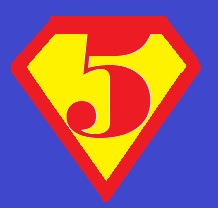Superman’s secret identity is Clark Kent, but did you know that the numbers 1, 2, 3, 4, and 5 also have secret identities? Before today, hardly anybody has known what their secret identities are, but I will reveal them to you now!

Let’s start with five. Its secret identity is zero. Five can change into a zero by smoothing its top and curving it down. When you count by fives you can see it changing into zero and back into five again as fast as Superman can change into Clark Kent.  5, 10, 15, 20, 25, 30, 35, 40, 45, 50, 55, 60, 65, 70, 75, 80, 85, 90, 95, 100, 105, and so forth.By using its secret identity , we can easily add or subtract five from a number ending in five or zero! 5 + 5 = 10, 10 + 5 = 15, and 15 – 5 = 10; 10 – 5 = 5.

Have you ever noticed how easy it is to turn a 4 into a 9?

That was as easy as Superman becoming Clark Kent!

When you count by 5’s starting at 4, these numbers turn into each other over and over again: 4, 9, 14, 19, 24, 29, and so forth. When you add 5 to either 4 or 9, it will turn into the other: 4 + 5 = 9, 9 + 5 = 14. The same thing happens when you subtract five: 14 – 5 = 9; 9 – 5 = 4. There isn’t any reason to count up or down; just remember how easy it is to turn a 4 into a 9 and vice versa.

A 3 can easily turn into an 8:• Counting by 5’s starting at 3 we get: 3, 8, 13, 18, 23, 28, and so forth.
• Also 3 + 5 = 8; 8 + 5 = 13; 13 – 5 = 8; 8 – 5 = 3.

If you cut off the tail at the bottom of a two, it can easily turn into its secret identity, seven:• Counting by 5’s starting at 2, we get: 2, 7, 12, 17, 22, 27, and so forth.
• 2 + 5 = 7; 7 + 5 = 12 and 12 – 5 = 7; 7 – 5 = 2.

Here’s how to discover the secret identity for the number 1. Take a strip of paper that looks like the number 1 and follow these SAFE directions for curling ribbon (or paper) with scissors. Curl the bottom half of the number 1 as well as the top fourth of that number 1 with scissors to make that number 1 look just like the number 6.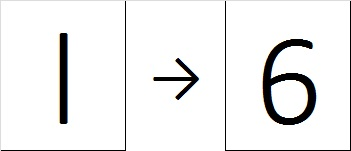• Counting by 5’s starting at 1, we get: 1, 6, 11, 16, 21, 26, and so forth.
• 1 + 5 = 6; 6 + 5 = 11 and 11 – 5 = 6; 6 – 5 = 1.

When Superman puts on glasses and a suit, we see his secret identity. Did you notice that odd digits have even secret identities and even digits have odd secret identities? Once a child memorizes these digits and their secret identities, he or she will be SUPER at adding or subtracting 5 and will never need to count up or down to get the answer.

Using these number transformations can help children memorize other addition facts. Once they know how to Add 1, 2, 3 and 4 and how to add 5, they can use that information to add 6, 7, 8, 9, or 10 by breaking up those numbers into a smaller number plus five. For example:

• 6 + 7 = 6 + (2 + 5) = (6 + 2) + 5 = 8 + 5 = 13.
• 7 + 9 = 7 + (4 + 5) = (7 + 4) + 5 = 11 + 5 = 16.

Learning facts for 6, 7, 8, 9, and 10 this way isn’t any more complicated than the common core approach[6 + 7 = 6 + (6 + 1) = (6 + 6) + 1 = 12 + 1 = 13], but is perhaps not as easy as just memorizing those facts and using flash cards or other means to drill them permanently into the brain. Secret identities and even “Flash” cards can be super fun.

# 111 and How Well Can You Add and Subtract in All Those Foreign Languages in Which You’ve Learned How to Count?

In general, adults understand how to add and subtract one-digit numbers, and most adults also have learned how to count in at least one language other than their native language. Does that mean these adults can add and subtract in those other languages in which they have learned how to count? I am curious to know the answer to that question. Let me tell you how counting, adding, and subtracting in a foreign language work for me.

I studied Spanish for three years in High School. I remember doing some basic addition and subtraction problems back then. Even though I remember very little Spanish vocabulary, I can still add and subtract one-digit numbers in Spanish fairly well.

I studied German for one and a half years in college. I remember even less German than I remember Spanish. All of the number words LOOK familiar, but I failed miserably when I tried to add and subtract using German numbers.

I lived in Turkey for two years when my husband was stationed at İncirlik Air Base near Adana. I learned a bit of vocabulary and how to count in Turkish. I actually remember the Turkish numbers a little better than the German ones, but my ability to add and subtract in Turkish isn’t much better.Now I am motivated to learn Hungarian. (I want to be able to understand my husband’s relatives better someday when I get to visit them in Hungary again.) The numbers in Hungarian are fresh in my mind, and I have even passed the time counting up to 1000 in Hungarian when I’ve gone for a walk.

When it came to doing basic addition or subtraction in Hungarian at this game site I found my ability to count did not help as much as I supposed. However, with PRACTICE, I have been able to greatly improve my ability to add and subtract in Hungarian.

After gaining the ability to add and subtract in Hungarian, I added another wrench to the process: I required myself to say complete number sentences in Hungarian instead of allowing English words like plus, minus, or equals to be used.From Hungarian Verbs & Essentials of Grammar, I learned that there are a few ways to say a number sentence when adding in Hungarian. The easiest two ways to say 4 + 3 = 7 are “Négy meg három (az) hét” or “Négy plusz három (az) hét.” Subtraction is a little more complicated because different numbers take two different case endings, ból or ből, in order to maintain vowel harmony. 10 – 4 = 6 is “Tízből négy az hat.” while 6 – 2 = 4 is “Hatból kettő az négy.”

Requiring myself to use complete Hungarian sentences has made me a little slower, and sometimes the hourglass empties before I click next to the correct number word. However, with PRACTICE I am getting better, and I will move on to the number games that use numbers up to 20 and 100 very soon.

This activity reinforces my belief that practice really does make a difference. I can see the merits of children practicing basic number facts in English or any other language they can speak. The FIND THE FACTORS puzzles are an excellent way for people of all ages to practice basic multiplication and division facts.

If you would like to see how well you can add and subtract using these learning games in other languages go to http://www.digitaldialects.com/.

Select one of the 70 available languages (listed in alphabetical order from left to right), select Numbers 1 -12, and begin the adding and subtracting game in the chosen language. I would love to know how well you do, so try it and leave a comment if your results don’t embarrass you too much!

Now I’ll tell you a little bit about the number 111:

111  is a composite number. 111 = 1 x 111 or 3 x 37. Factors of 111: 1, 3, 37, 111. Prime Factorization: 111 = 3 x 37.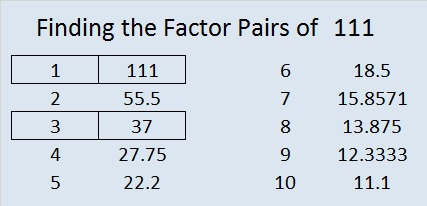111 is never a clue in the FIND THE FACTORS puzzles.

111 = (1 + 2 + 3 + . . . + 35 +36)/6, which can be more easily calculated by using 111 = (36 x 37)/(2 x 6). Therefore, 111 is the magic sum of any 6 x 6 magic square that contains all the integers from 1 to 36.